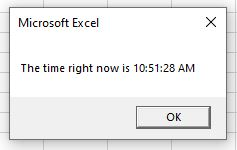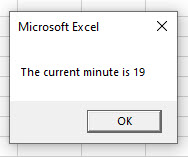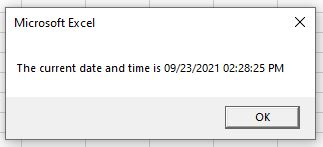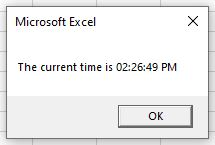# VBA Time Functions

This tutorial will demonstrate how to use VBA Time Functions.

Dates and Time in Excel are stored as numbers, and then formatted to show as a date. Day 1 in the world of Excel was the 1st January 1900 (Windows default) or 1st January 1904 (Macintosh default) – which means that the 5th August 2021 is day 44413 since the 1st January 1900.  To use the Time functions in Excel, we need to include decimal points in the number, so for example, 44413.456 is 10.56am on the 5th August 2021 where 44413.5 will be exactly 12 noon.

## Time Function

The VBA Time Function does not require any arguments.  The syntax is simply Time()

``````Sub GetCurrentTime()
MsgBox "The time right now is " & Time()
End Sub``````

Running the above procedure will return the following message to the user:## Hour Function

The Hour Function in VBA will return the hour part of the time inputted.

``````Sub GetHour()
MsgBox "The current hour is " & Hour(Time())
End Sub``````

The procedure above combines the Time() and Hour() functions to give us the current hour of the day.

We  can also input the time, and the hour for that time will be returned.

``````Sub GetHour()
MsgBox "The current hour is " & Hour("5:25:16 AM")
End Sub``````

## Minute Function

As with the Hour Function, the Minute function will return the minute part of the time inputted.

``````Sub GetMinute()
MsgBox "The current minute is " & Minute(Time())
End Sub``````

Similarly, we can input the time, and the minute will be returned.

``````Sub GetMinute()
MsgBox "The current minute is " & Minute("5:25:16 AM")
End Sub``````

We can also input the time as a serial number and get the minute or hour from the decimal amount.

``````Sub GetMinute()
MsgBox "The current minute is " & Minute(44651.597)
End Sub
``````## Second Function

The second function returns the second part of the time inputted in the same way as the hour and minute functions.

``````Sub GetSecond()
MsgBox "The current second is " & Minute(Time())
End Sub``````

## Now Function

The Now() function returns the current date and time

``````Sub GetNow()
MsgBox "The current date and time is " & Now()
End Sub``````To just get the time from the Now() function, we can use the Format() Function to format the returned value just to show the time.

``````Sub GetNow()
MsgBox "The current time is " & Format(Now(), "hh:mm:ss AMPM")
End Sub``````Stop searching for VBA code online. Learn more about AutoMacro - A VBA Code Builder that allows beginners to code procedures from scratch with minimal coding knowledge and with many time-saving features for all users!